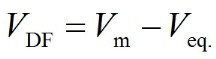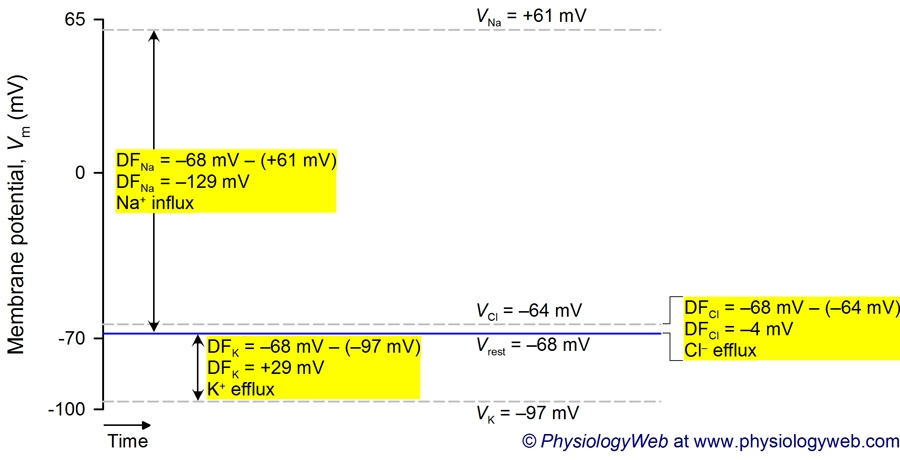PhysiologyWeb  Home  |  FAQ  |  About  |  Contact  |   - Sign InResting Membrane Potential -
Electrochemical Driving Force Acting on Ions
In the previous section, we discussed how the membrane potential can be calculated by using the Goldman-Hodgkin-Katz (GHK) equation. We also discussed how changes in the relative permeability values for K+ (pK), Na+ (pNa), and Cl (pCl) can lead to changes in the membrane potential (see figure). In this section, we wish to better understand the relationship between the membrane potential (Vm) and the equilibrium potential (Veq.) for the ions that contribute to the membrane potential. We will see that if the membrane potential is not exactly at the equilibrium potential for an ion, then an electrochemical driving force acts on the ion which causes the ion to flow into or out of the cell (see below for details).
When two or more ions contribute to the membrane potential across the plasma membrane (Vm) of a cell, it is likely that the membrane potential would not be at the equilibrium potential (Veq.) for any of the contributing ions. Thus, no ion would be at its equilibrium (i.e., Veq.Vm). For example, the resting membrane potential of a typical neuron is neither at the K+, Na+, nor Cl equilibrium potential (see figure). This means that at the resting potential, K+, Na+, and Cl are not at electrochemical equilibrium and, thus, the chemical and electrical forces acting on K+, Na+, and Cl are not equal. When an ion is not at its equilibrium, an electrochemical driving force (VDF) acts on the ion, causing the net movement of the ion across the membrane down its own electrochemical gradient. The driving force is quantified by the difference between the membrane potential and the ion equilibrium potential (VDF = VmVeq.). The driving force is the net electromotive force that acts on the ion. The magnitude of the driving force indicates how far the membrane potential (Vm) is from the electrochemical equilibrium (Veq.) of an ion. Thus, the magnitude of the driving force indicates how far an ion is from its equilibrium. The arithmetic sign (i.e., positive or negative) of the driving force acting on an ion along with the knowledge of the valence of the ion (i.e., cation or anion) can be used to predict the direction of ion flow across the plasma membrane (i.e., into or out of the cell). See Table 1 below.Eq. 1
• VDF is the electrochemical driving force acting on the ion of interest. Its value is generally reported in millivolts (mV). Its arithmetic sign (i.e., positive or negative) along with the knowledge of the valence of the ion (i.e., cation or anion) can be used to predict the direction of ion flow across the plasma membrane (i.e., into or out of the cell). Our online Electrochemical Driving Force calculator can be used to perform calculations involving the electrochemical driving force.
• Vm is the membrane potential. The membrane potential may be obtained by direct measurement, or may be predicted by using the Goldman-Hodgkin-Katz (GHK) equation.
• Veq. is the equilibrium potential for the ion of interest. Its value may be determined by using the Nernst equation.

Table 1. Sign of electrochemical driving force (VDF) and direction of ion flow
 Ionic species Sign of driving force(VDF = Vm − Veq.) Direction of ion flow Cation +0− OutwardNo net flowInward Anion +0− InwardNo net flowOutward
+ refers to VDF > 0, refers to VDF < 0, and 0 refers to VDF = 0. When VDF = 0, the ion is in electrochemical equilibrium. Cation, positively-charged ion; Anion, negatively-charged ion; Outward, ion movement out of the cell; Inward, ion movement into the cell.
Table 1 summarizes how the arithmetic sign of the driving force may be used to predict the direction of ion flow across the plasma membrane (i.e., into or out of the cell). For cations (positively charged ions such as Na+, K+, H+, and Ca2+), a positive driving force (i.e., VDF > 0) predicts ion movement out of the cell (efflux) down the ion's electrochemical gradient, and a negative driving force (i.e., VDF < 0) predicts ion movement into the cell (influx). The situation is reversed for anions (negatively charged ions such as Cl and HCO3), where a positive driving force predicts ion movement into the cell (influx), and a negative driving force predicts ion movement out of the cell (efflux). If the membrane potential (Vm) is exactly at the equilibrium potential (Veq.) for an ion (Vm = Veq.), the driving force acting on the ion would be zero (VDF = 0). If Vm = Veq., it can be seen that VDF = VmVeq. = 0. In this case, the ion is said to be in electrochemical equilibrium, and there would be no net movement of the ion across the plasma membrane into or out of the cell (i.e., no net flux of ion).
Using the information provided in Table 1 and the equilibrium potential for any given ionic species, you should be able to determine the direction of flow for that ion at a given membrane potential. For example, assume that VK = −90 mV, and Vm = −65 mV. Thus, the driving force acting on K+ is: DFK = −65 − −90 = +25 mV. The sign of the driving force is positive. Since K+ is a positively charged ion (cation), a positive value for the driving force indicates that K+ is flowing out of the cell (under these conditions).
As a second example, assume that VCl = −65 mV. Using the same reasoning, you should be able to conclude that if Vm = −65 mV, there would be no net movement of Cl into or out of the cell. The driving force acting on Cl here is DFCl = −65 − −65 = 0 mV. It should be intuitive that if the membrane potential is at the equilibrium potential for an ion, no net force acts on the ion (i.e., driving force = 0), and there will be no net movement of the ion across the plasma membrane. Indeed, in many cells, the contribution of Cl to the membrane potential is small and VCl usually (but not always) follows Vm (i.e., Cl distributes across the membrane to adjust the inside concentration to establish a VCl that is close to Vm). While in these cases, Cl may not contribute greatly to the resting membrane potential, the existing Cl permeability does guard against large changes in the membrane potential. As we will see in future lectures, this is extremely important in excitable cells such as neurons.Figure 1. Graphical representation of the electrochemical driving force acting on potassium, sodium, and chloride ions for a neuron at rest.
This figure demonstrates a graphical representation of the electrochemical driving force that acts on potassium, sodium, and chloride ions for a neuron at rest. It also indicates the direction of ion flow based on the sign of the driving force. Driving force is calculated for each ion according to VDF = VmVeq. (see electrochemical driving force calculator). Vm is calculated by using the Goldman-Hodgkin-Katz equation. Veq. is calculated for each ion by using the Nernst equation.
You should use the electrochemical driving force calculator to perform additional calculations for K+, Na+, and Cl at a variety of membrane potentials until it becomes second nature to you and you begin to intuitively appreciate how the driving force influences the net movement of cations and anions across the plasma membrane.
 Previous: In Real Cells, Multiple Ions Contribute to the Membrane Potential Next: Maintenance of the Membrane Potential

Posted: Saturday, February 15, 2014